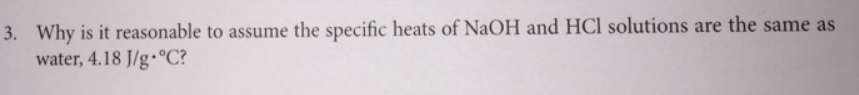Chemistry Calorimetry Solution: Why is it reasonable to assume the specific heats ...

# Solution: Why is it reasonable to assume the specific heats of NaOH and HCl solutions are the same as water, 4.18 J/g•°C?

Problem

Why is it reasonable to assume the specific heats of NaOH and HCl solutions are the same as water, 4.18 J/g•°C?View Complete Written Solution